# C Programming Tutorial for Beginners

## 1- Introduction

This document is based on:

## 2- What is needed to get started with C/C++?

We use Eclipse IDE for documents which guide about  C/C++ on  o7planning  website. You need to setup and configure  Eclipse and C/C++ compiler before starting. You can see instruction at:

## 3- Differentiating C and C++

You need an overview to recognize the C and C++.
C is predecessors language and the procedure-oriented language, it is easy to be deployed and run on the operating systems. C++ was born after expanding from C and it brings in the concept of object-oriented programming, C is the foundation of C++, and C++ are not born to replace C,  its libraries is expanded tremendously.
Procedure-oriented means: The source files (Containing your code) will contain the functions. Meanwhile object-oriented  source file contains a class in which  contains methods. To call a method in a class you need to create an instance of the class, then call the method through this object, whereas with procedure-oriented you can call function directly.
In this guide I will show you how to work on C. But C++ will be discussed in a separate document.

If you work with:  Windows 64bit + Eclipse 64bit + Java64bit, you need to open Eclipse with Administrator right, there is trouble that  Eclipse does not print the message on the   Console screen in case of running in normal mode.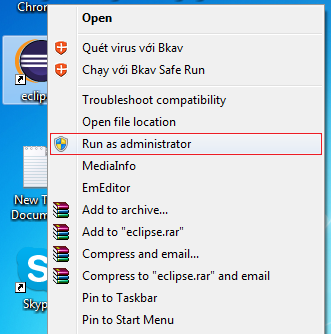• File/New/Other..
In this document, I will guide you how to program C (C++ will be guided in other document). Although project that we create here is C++, but we only use libraries of C.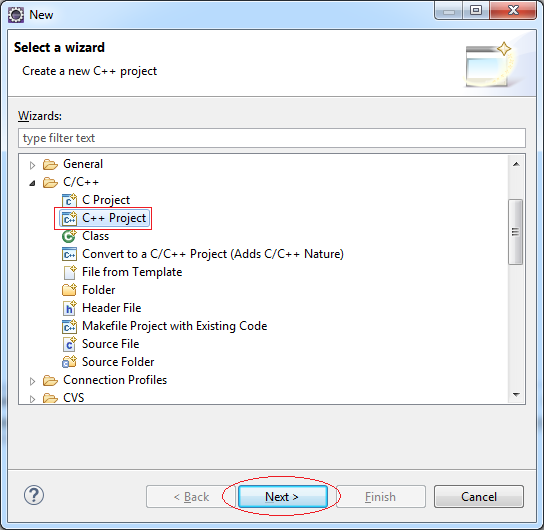Enter the name of the project:
• CBasicTutorial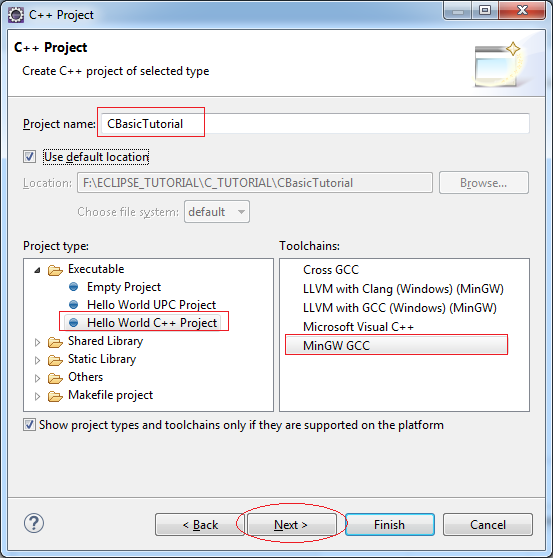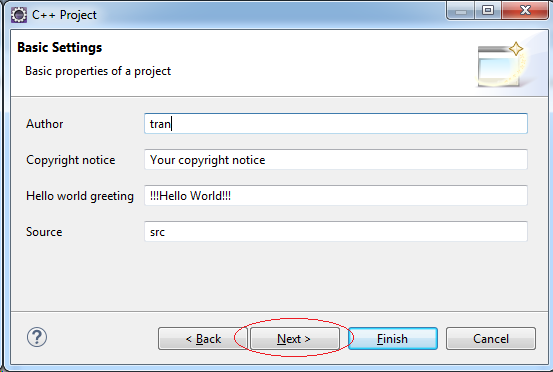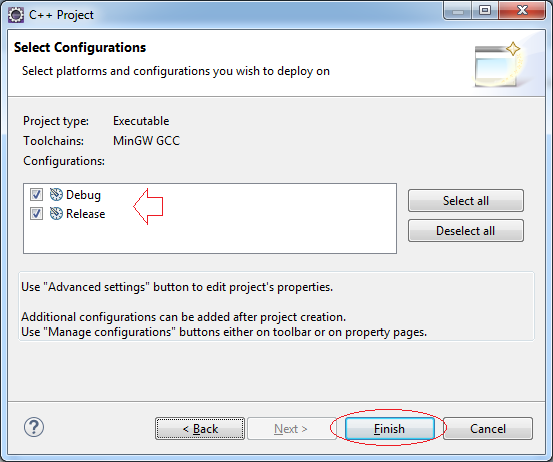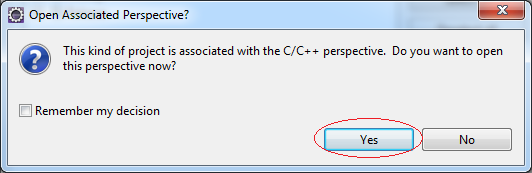Project was created, actually code of  HelloWorld is C++ . But there is no need to concern about it.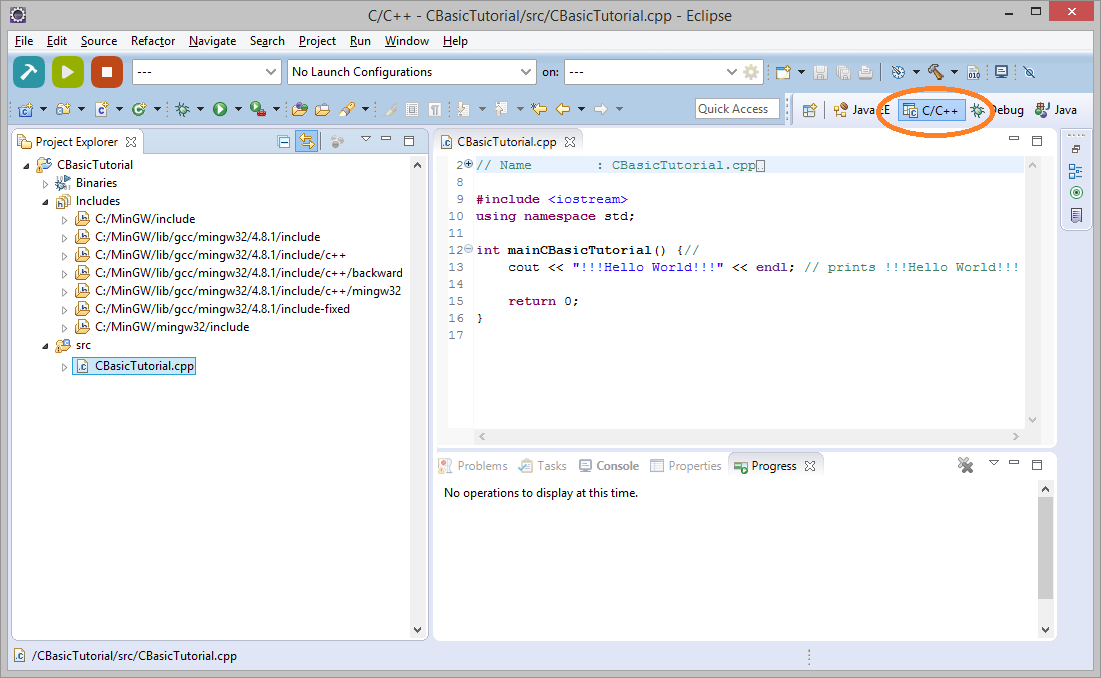Compile the project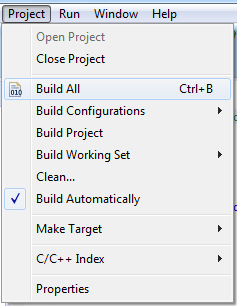Project has been successfully compiled.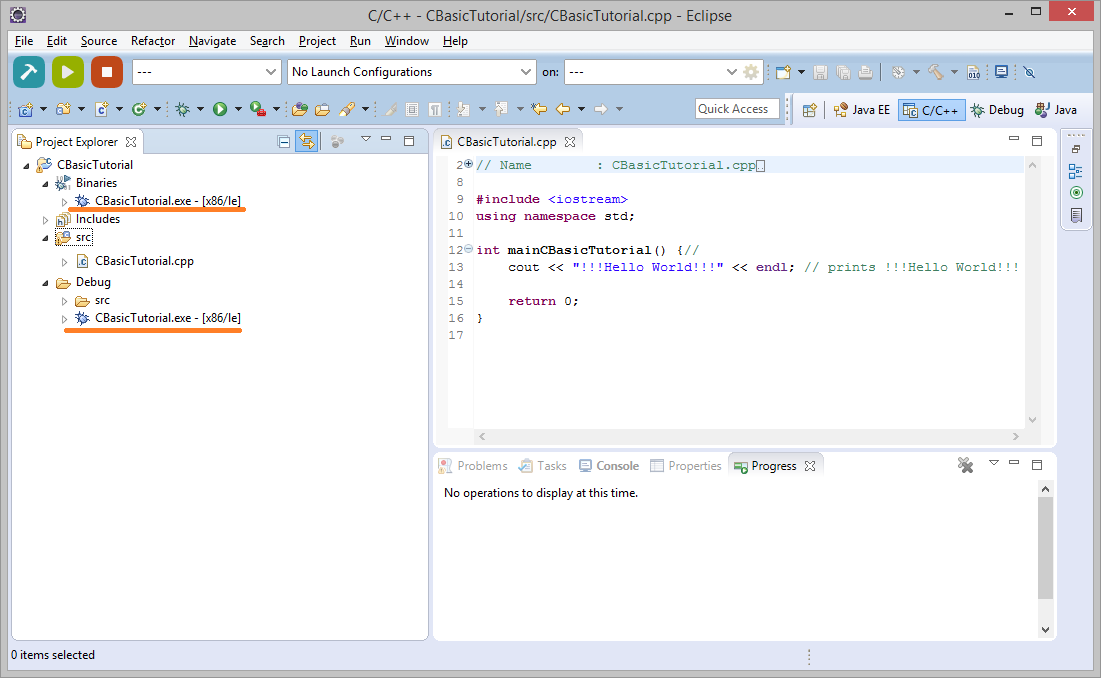Next you need to configure to  run project directly on the Eclipse, it is very important.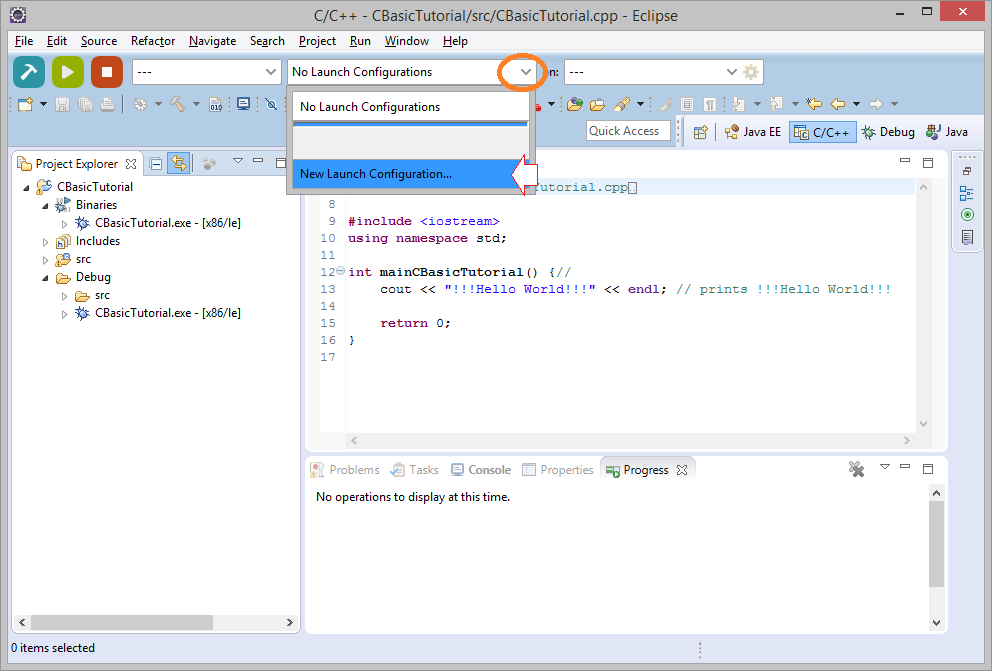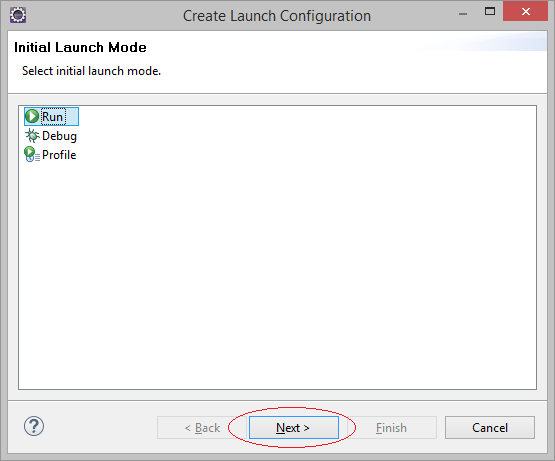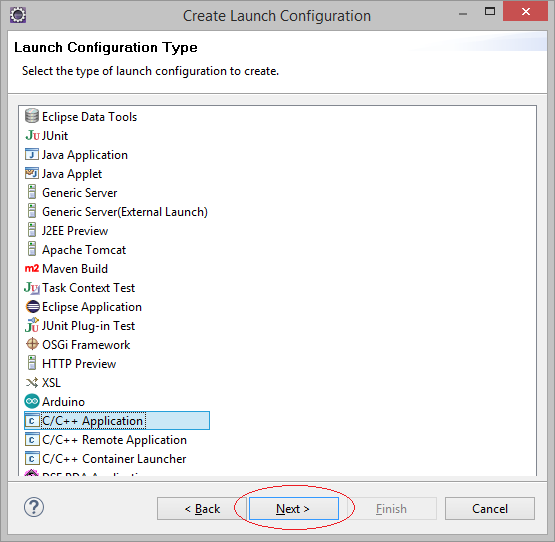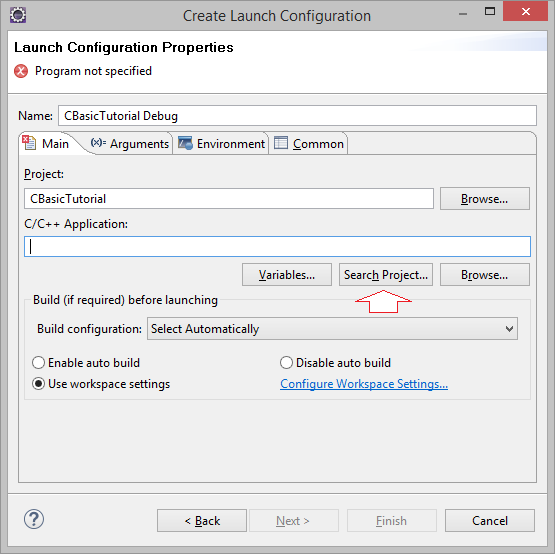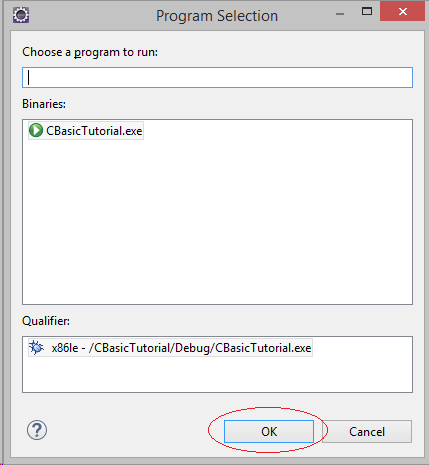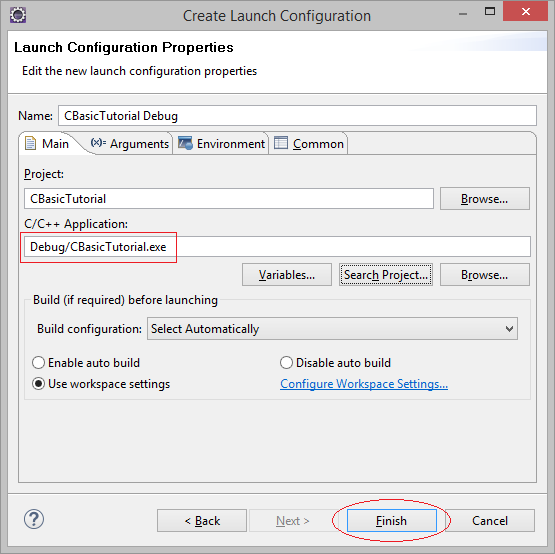Running HelloWorld example: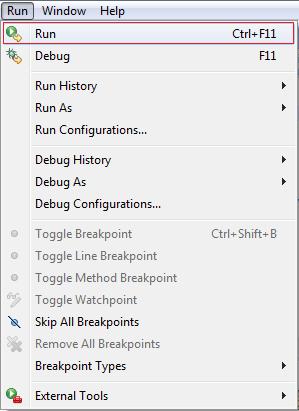OR: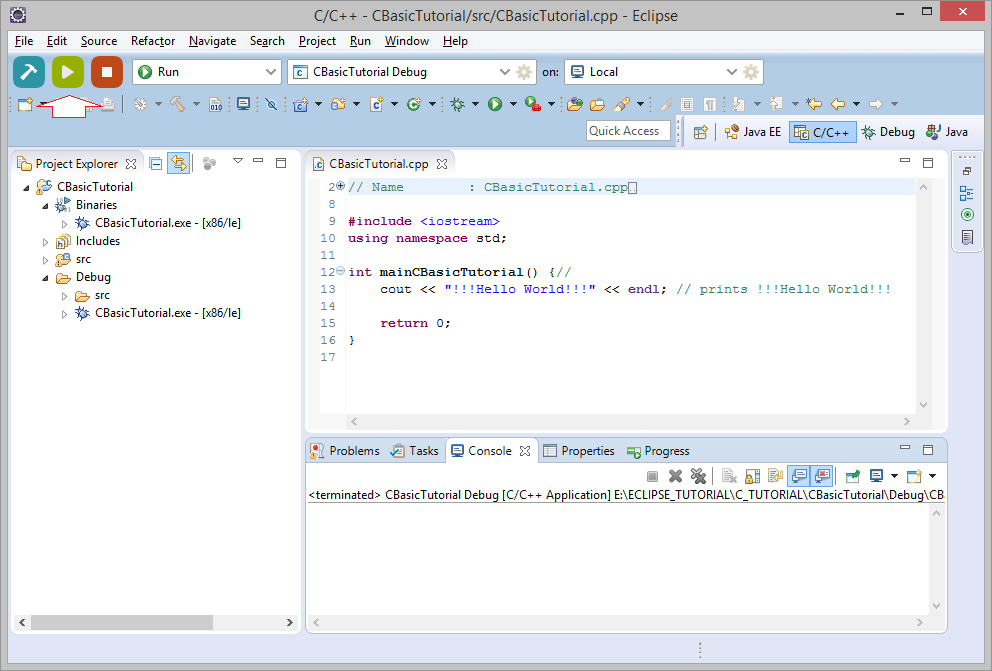Results:OK! Everything works fine.

## 5- The characteristics of C and attention in practice

When a C program is run, it will find main() function to execute, such as executing main() function of the above  HelloWorld example.

A C program can have multiple  source file, each source file can have many functions. However, it  allows only one main() function on your entire Project.
Above there, you just created a CBasicTutorial.cpp with a main() function, you now create another file to practice, such as PrimitiveExample.cpp and main() function that you need to rename the main() function of  CBasicTutorial.cpp so it does not conflict and you can compile it, then can practice what is written in PrimitiveExample.cpp.
In my opinion,when practicing you should choose to rename the following:
• CBasicTutorial.cpp
• main()  ==> mainCBasicTutorial()

## 6- The structure of a C program

I will create a cpp file to illustrate and explain about structure of C program.
In Eclipse, select:
• File/New/Other...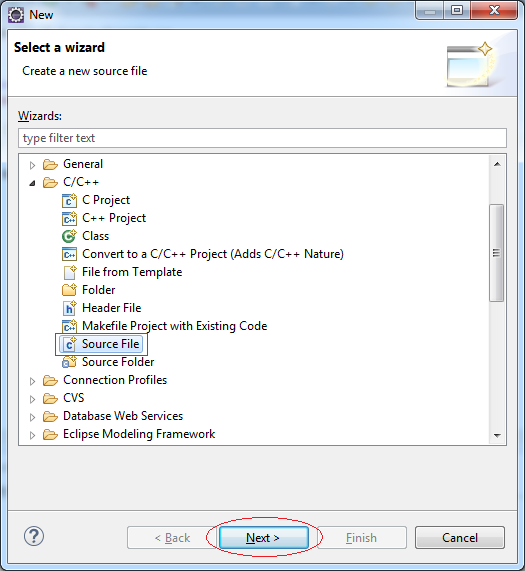Enter:
• Source file: MyFirstExample.cpp
• Template: Default C source temple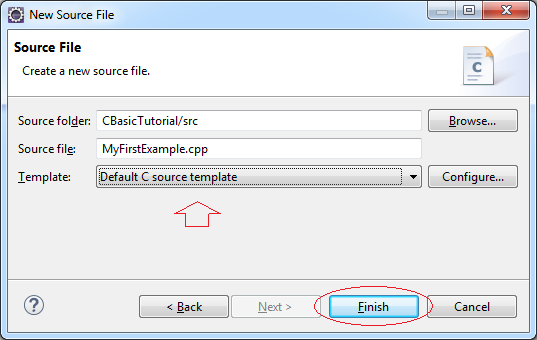Source file was created, there is nothing.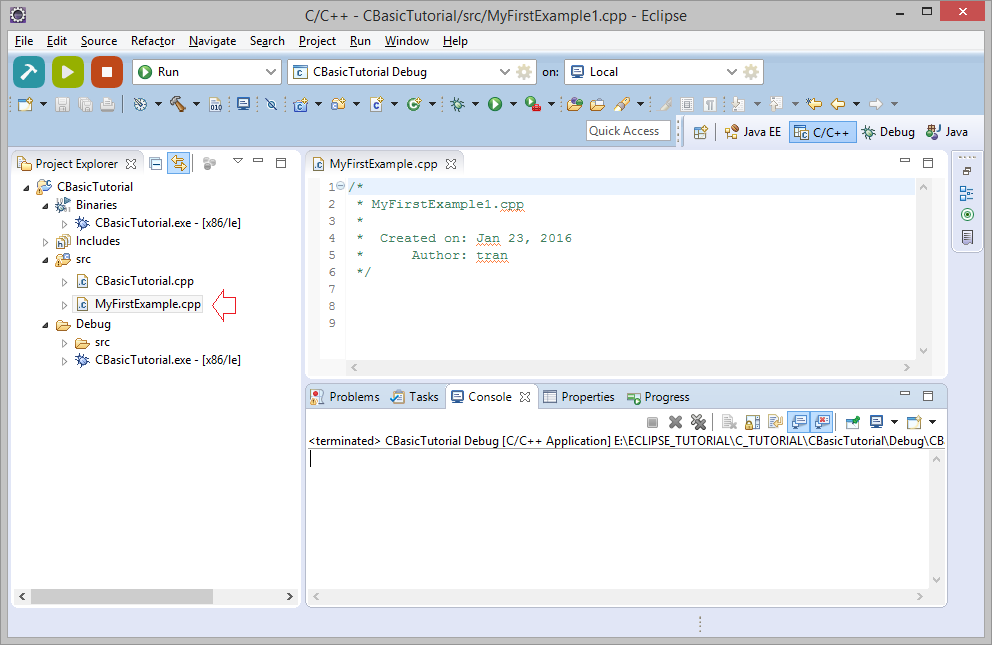We will write the code for this source file:
Note: Change the name of the main function of CBasicTutorial.cpp source file into mainCBasicTutorial because C project  only allows a main function across the entire Project.
MyFirstExample.cpp
```// Declare for use the standard IO library stdio.h.
#include<stdio.h>

int main() {

// printf is a function of the library stdio.h
// This is a function to print out a text to console.
// \n is the newline character.
printf("Hello!, This is your first C example.\n");

// Print out the message, the application will end.
printf("Exit!");

// This Function returns 0.
return 0;

}
```
Running the example: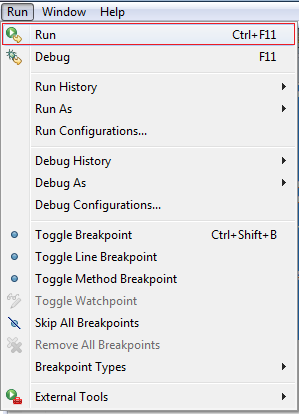Results: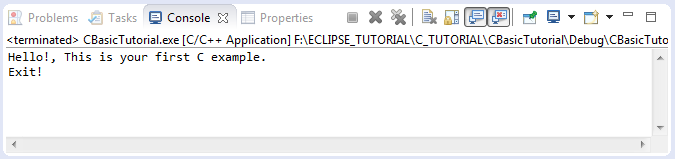Note: There are some functions in conio.h libraries of C are not supported in C ++. Such as getch() - which originally is a function to pause program and waiting until the user types a new character to continue to run. So in this tutorial I try not to use such function in the examples.

```#include<conio.h>

int main() {

// Do something here ....
// Stop program here, wait until the user presses any character.
// (This function is not fully supported)
getch();

// Do something here.
}
```

## 7- Data types in C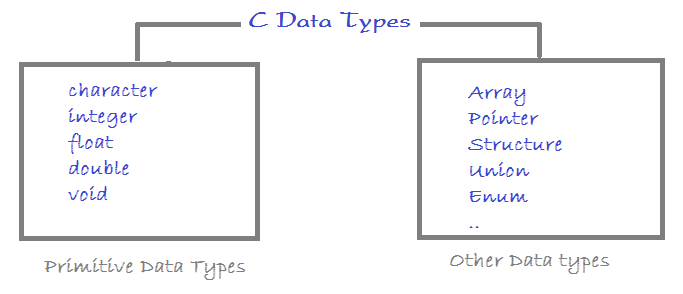### 7.1- Integer data types

Type Storage size Value range Format
char 1 byte -128 to 127 or 0 to 255 %c
unsigned char 1 byte 0 to 255 %c
signed char 1 byte -128 to 127 %s
int 2 or 4 bytes -32,768 to 32,767 or -2,147,483,648 to 2,147,483,647 %d
unsigned int 2 or 4 bytes 0 to 65,535 or 0 to 4,294,967,295 %u
short 2 bytes -32,768 to 32,767
unsigned short 2 bytes 0 to 65,535
long 4 bytes -2,147,483,648 to 2,147,483,647 %ld
unsigned long 4 bytes 0 to 4,294,967,295

### 7.2- Floating point type

Type Storage size Value range Precision
float 4 byte 1.2E-38 to 3.4E+38 6 decimal places
double 8 byte 2.3E-308 to 1.7E+308 15 decimal places
long double 10 byte 3.4E-4932 to 1.1E+4932 19 decimal places

### 7.3- Example

PrimitiveExample.cpp
```// Declare for use the standard IO library stdio.h.
#include <stdio.h>

// Declare for use float.h library.
#include <float.h>

int main() {

// The sizeof(type) function
// returns the number of bytes to store data types
printf("Storage size for float : %d \n", sizeof(float));

// FLT_MIN is constant, the minimum value of float data type.
// This constant is defined in the library float.h
printf("Minimum float positive value: %E\n", FLT_MIN);

// FLT_MAX is constant, the maximum value of float data type.
// This constant is defined in the library float.h
printf("Maximum float positive value: %E\n", FLT_MAX);

// FLT_DIG is constant, the maximum number of decimal fraction.
// This constant is defined in the library float.h
printf("Precision value: %d\n", FLT_DIG);

return 0;

}
```
Running the example: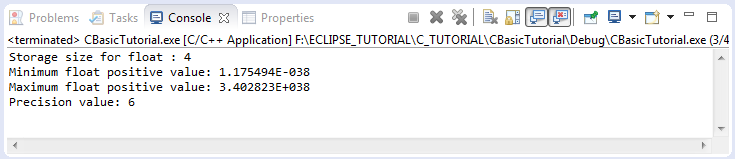## 8- Branch statement in C (if - else if - else)

if is a command which is used to check condition in C. For example: If a > b, do something....
The common comparison operators:
 Operator Meaning Example > greater than 5 > 4 is true < less than 4 < 5 is true >= greater than or equal 4 >= 4 is true <= less than or equal 3 <= 4 is true == equal to 1 == 1 is true != not equal to 1 != 2 is true
```// Syntax
if ( condition ) {

// Do something here
}
```
Example:
```// Example 1:
if ( 5 < 10 ) {
printf( "Five is now less than ten");
}

// Example 2:
if ( true ) {
printf( "Do something here");
}
```
Full structure of the If-Else if-Else:
```// Note that there will be only at most one block can be run.
// Program tests conditions from top to bottom.
// When meeting a condition in which the correct block will be run,
// and the program does not check to the remaining conditions.
...

// If condition 1 is true then ...
if ( condition1) {

// Do something when condition 1 is true.
}
// Else if condition 2 is true, then ...
else if( condition2 ) {

// Do something when condition 2 is true
// (Condition 1 is false).
}
// Else if condition N is true, then ...
else if( conditionN ) {
// Do something when condition 2 is true
// (The above conditions are false).
}
// The remaining cases.
else {
// Do something here.
}
```
Example:
IfElseExample.cpp
```// Declare to use standard IO Library.
#include <stdio.h>

int main_IfElseExample() {

// Declare a number representing your age.
int age;

// Sometimes printf does not print out your message to Console immediately.
// Using  fflush(stdout) to print out message to Console immediately.
// Note: stdout is a variable of stream which is printed out the screen.
// (It is defined in the stdio.h library)
fflush (stdout);

// scanf function will wait you type into a text from the keyboard
// (And press Enter to complete).
// It will scan to take a number (Specify by %d parameter)
// and assign to the age variable.
scanf("%d", &age);

// Check if age is less than 80 then ...
if (age < 80) {
printf("You are pretty young");
}

// Else if the age is between 80 and 100 then
else if (age >= 80 && age <= 100) {
printf("You are old");
}
// Else (The remaining cases)
else {
printf("You are verry old");
}

return 0;
}
```
Running the example: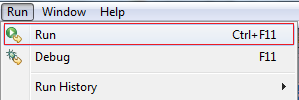The program prints out text which ask you to enter a number, type 70 and press Enter.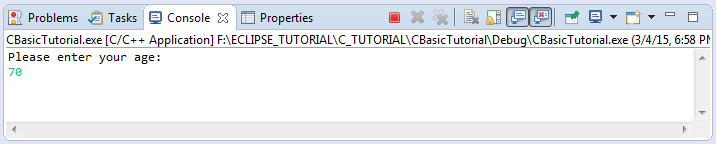Results: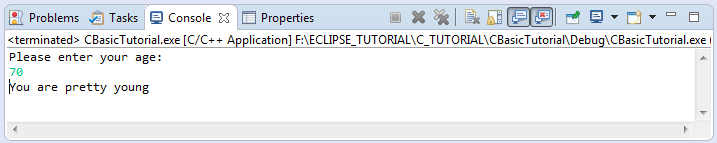You can run the example  again and enter the other numbers and see the result.

## 9- switch statement

Syntax of switch statement:
```// Use the switch to check the value of a variable.
switch ( a_variable ) {
case value1:
// Do something here, if a_variable == value1
break;
case value2:
// Do something here, if a_variable == value2
break;

default:
// Do something here
// if the value of the variable is different from the values listed above.
break;
}
```
SwitchExample.cpp
```#include <stdio.h>

int main() {

// Asks the user to choose one option.

printf("1 - Play a game \n");
printf("2 - Play music \n");
printf("3 - Shutdown computer \n");

fflush (stdout);

// Declare an variable.
int option;

// scanf function will wait for user to enter text from the keyboard
// (And press enter to complete).
// It will scan to get a number (Specify by parameter %d)
// And convert to int and asign to 'option' variable.
scanf("%d", &option);

// Check the value of 'option'.
switch (option) {

case 1:
printf("You choose to play the game \n");
break;
case 2:
printf("You choose to play the music \n");
break;
case 3:
printf("You choose to shutdown the computer \n");
break;
default:
printf("Nothing to do...\n");
break;
}

fflush(stdout);

return 0;

}
```
The result when running the example (in case enter 2 and press Enter).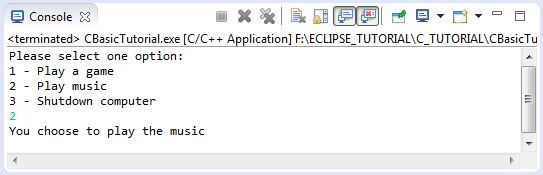## Note:

You wonder, 'break' in the block of 'case' mean?

break in this case told the program that exit switch. In case you do not use break, the program will continue to execute blocks in the 'case' below (and default block) until caught break, including the value of the variable in that case is different from the value of ' case'.
Consider an illustration:
SwitchExample2.cpp
```#include <stdio.h>

int main() {

// Declare variable 'option' and assigns the value of 3.
int option = 3;

printf("Option = %d \n", option);

// Check the value of 'option'
switch (option) {

case 1:
printf("Case 1 \n");
break;
case 2:
printf("Case 2 \n");
// No break
case 3:
printf("Case 3 \n");
// No break
case 4:
printf("Case 4 \n");
// No break
case 5:
printf("Case 5!!! \n");
break;
default:
printf("Nothing to do...\n");
break;
}

fflush (stdout);

return 0;

}
```
Running the example: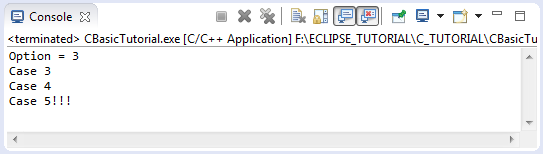## 10- The loop in C

Loops are used to repeat a block of code. Being able to have your program repeatedly execute a block of code is one of the most basic but useful tasks in programming
C hỗ trợ 3 loại vòng lặp khác nhau:
• FOR
• WHILE
• DO WHILE

### 10.1- FOR loop

The syntax of the FOR loop:
```
for ( variable_initialization; condition; variable_update ) {
// Code to execute while the condition is true.
}
```
Example:
```// Example 1:
// Create variable x and assign value of 0 to it.
// Check condition is x < 5.
// If x < 5 is true then execute this block.
// Increase x by 1 after each iteration.
for (int x = 0; x < 5; x = x + 1) {
// Do something here when x < 5 is true.
}

// Example 2:
// Create variable x and assign value of 2 to it.
// Check condition is x < 15
// If x < 15 is true then execute this block.
// Increase x by 3 after each loop.
for (int x = 2; x < 15; x = x + 3) {
// Do something here when x < 15 is true.
}
```
ForLoopExample.cpp
```#include <stdio.h>

int main() {

printf("For loop example\n");

// Sometimes printf does not print out your message to Console immediately.
// Using  fflush(stdout) to print out message to Console immediately.
// Note: stdout is a variable of stream which is printed out the Console.
// (It is defined in the stdio.h library).
fflush (stdout);

// Declare variable x and assign value of 2.
// Check condition is x <15
// If x < 15 is true, then run this block.
// Increase the value of x by 3 after each iteration.
for (int x = 2; x < 15; x = x + 3) {
printf("\n");
printf("Value of x = %d \n", x);
fflush(stdout);
}
return 0;

}
```
Running the example: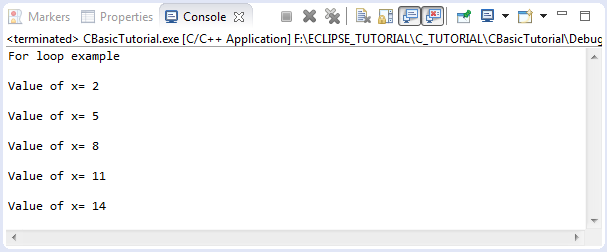### 10.2- WHILE loop

Syntax of WHILE loop:
```while ( condition ) {

// When condition is true then execute the block.
}
```
Example:
```// Example 1:
// Declare a variable x.
int x = 2;

while ( x < 10) {
// Do something here when x < 10 is true.
// ....
// Update new value to x.
x = x + 3;
}
```
WhileLoopExample.cpp
```#include <stdio.h>

int main() {

printf("While loop example\n");

fflush (stdout);

// Declare variable x and assign value of 2.
int x = 2;

// Check condition is x < 10.
// If x < 10 then run the block.
while (x < 10) {
printf("Value of x = %d \n", x);

x = x + 3;
fflush(stdout);
}
return 0;

}
```
Running the example: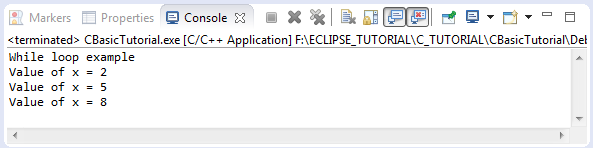### 10.3- DO WHILE loop

Syntax of DO-WHILE loop:
```// The characteristics of 'do-while' loop is that it execute block at least 1 time.
// After each iteration it checks the conditions for the next iteration.
do {

// Do something here.
}while ( condition );  // Note: Need ';'.
```
DoWhileLoopExample.cpp
```#include <stdio.h>

int main() {

printf("Do-While loop example\n");

fflush (stdout);

// Create variable x and assign value of 2.
int x = 2;

// The characteristics of 'do-while' loop is that it execute block at least 1 time.
// Then check conditions whether continuing to run this block or not.
do {
printf("Value of x = %d \n", x);

x = x + 3;
fflush(stdout);
} while (x < 10); // Note: ==> Need ';'

return 0;

}
```
Running the example: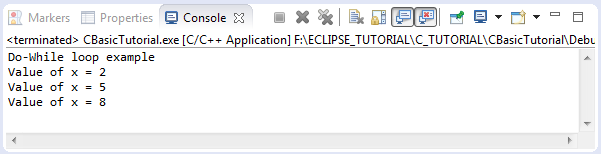### 10.4- break statement within the loop

break is a command which can be included in block of  loop. This is the command to end loop unconditionally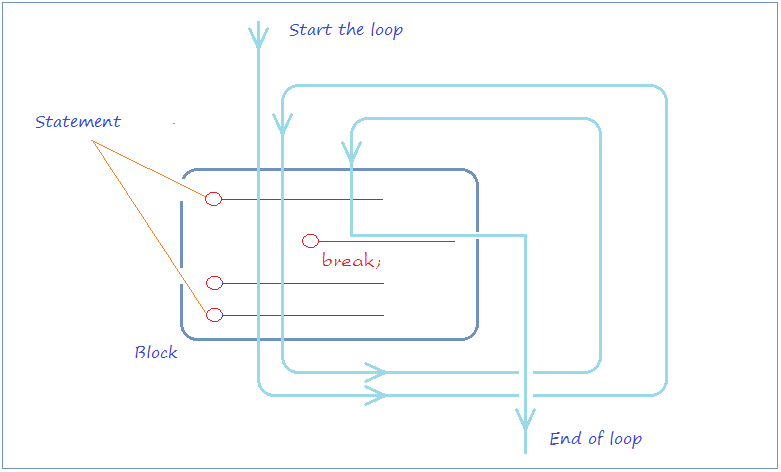BreakExample.cpp
```#include <stdio.h>

int main() {

printf("Break example\n");

fflush (stdout);

// Create a variable x and assigns the value of 2.
int x = 2;

while (x < 15) {

printf("----------------------\n");
printf("x = %d \n", x);

// Check if x = 5, then exit the the loop.
if (x == 5) {
break;
}
// Increasing x by 1 (Short for x = x + 1).
x++;
printf("x after ++ = %d \n", x);

}
return 0;

}
```
Running the example: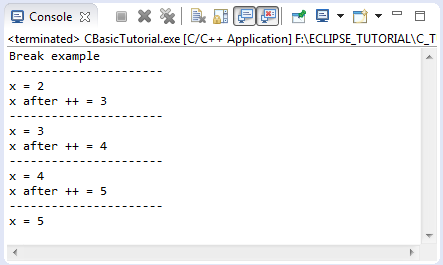### 10.5- continue statement within the loop

continue is a command which can be included in a loop, when caught continue command, the program will ignore the command line in the block and under the continue and start a new loop.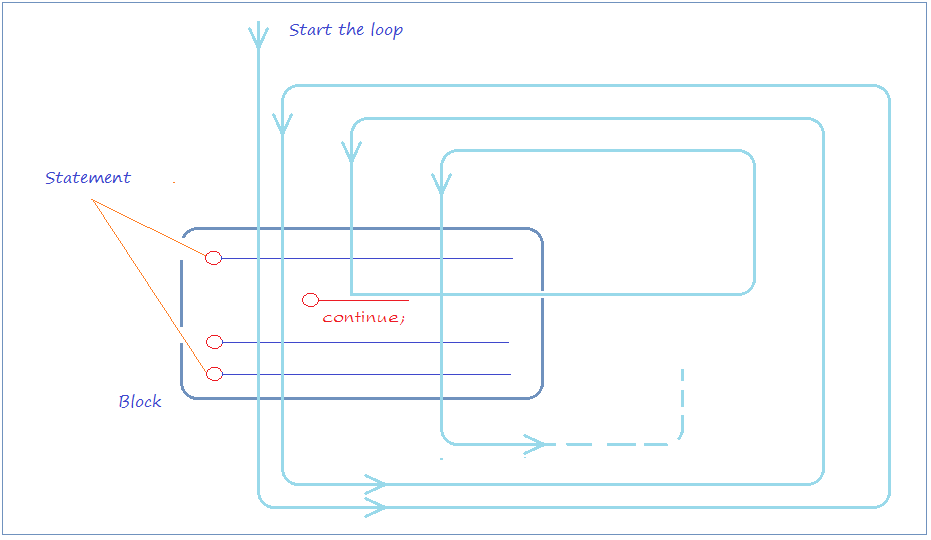ContinueExample.cpp
```#include <stdio.h>

int main() {

printf("Continue example\n");

fflush (stdout);

// Create variable x, and assign value of 2.
int x = 2;

while (x < 7) {

printf("----------------------\n");
printf("x = %d \n", x);

// % is operator returns remainder.
// If x is even, then ignore the below line of the 'continue',
// to continue the new iteration (if any).
if (x % 2 == 0) {
// Increase x by 1 (Short for x = x + 1).
x++;
continue;
} else {
// Increase x by 1 (Short for x = x + 1).
x++;
}
printf("x after ++ = %d \n", x);

}
return 0;

}
```
Running the example: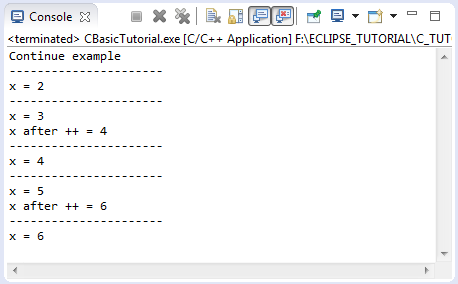## 11- Arrays in C

### 11.1- One-dimensional array

These are illustrations of one-dimensional array with 5 elements, the elements are indexed from 0 to 4.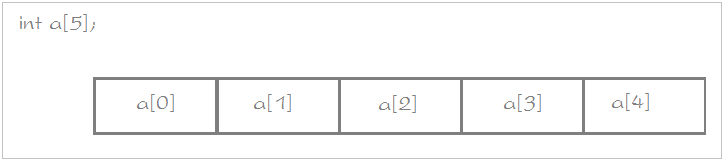The syntax to declare a one-dimensional array:
```// Way 1:
// Declare an array of int numbers, specifying the elements.
int years[] = { 2001, 2003, 2005, 1980, 2003 };

// Way 2:
// Declare an array of 5 elements,
// specify the value for the first 3 elements.
int age = { 20, 10, 50 };

// Way 3:
// Declare an array of floats, specifying the number of elements.
// (Size of 3)
float salaries;
```
ArrayExample1.cpp
```#include <stdio.h>

int main() {

// Way 1:
// Declare an array with elements.
int years[] = { 2001, 2003, 2005, 1980, 2003 };

// Print out size in bytes of int type.
printf("Sizeof(int) = %d \n", sizeof(int));

// Size in bytes of array
printf("Sizeof(years) = %d \n", sizeof(years));

int arrayLength = sizeof(years) / sizeof(int);

printf("Element count of array years = %d \n\n", arrayLength);

// Use a for loop to print out the elements of the array.
for (int i = 0; i < arrayLength; i++) {
printf("Element at %d = %d \n", i, years[i]);
}

fflush (stdout);

// Way 2:
// Declare an array of size 3.
float salaries;

// Assign values to the elements.
salaries = 1000;
salaries = 1200;
salaries = 1100;

return 0;

}
```
Running the example: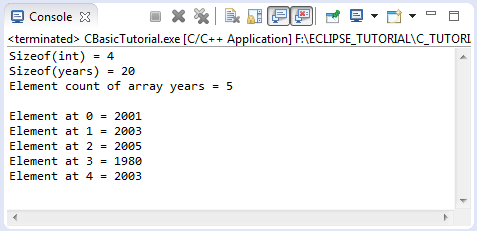### 11.2- Two-dimensional array

This is illustrated a two-dimensional array: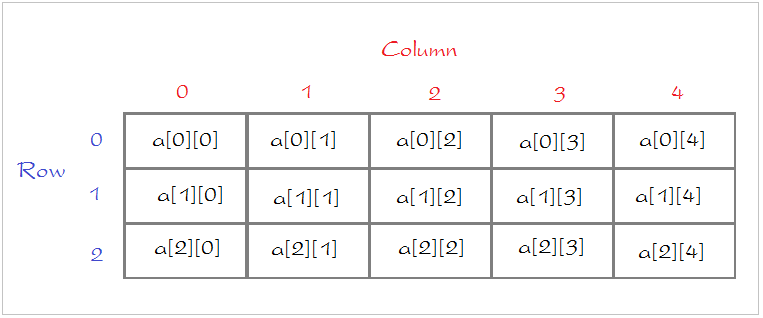The syntax to declare a two-dimensional array:
```// Declare a two-dimensional array.
// (3 rows and 5 columns)
int a = { { 1, 2, 3, 4, 5 },
{ 0, 3, 4, 5, 7 },
{ 0, 3, 4, 0, 0 } };

// Declare an array,
// specify the number of rows, number of columns
int a;
```
ArrayExample2.cpp
```#include <stdio.h>

int main() {

// Declare a two-dimensional array (3 rows and 5 columns)
int a = { { 1, 2, 3, 4, 5 }, { 0, 3, 4, 5, 7 }, { 0, 3, 4, 0, 0 } };

// Use a for loop to print out the elements of the array.
for (int row = 0; row < 3; row++) {
for (int col = 0; col < 5; col++) {
printf("Element at [%d,%d] = %d \n", row, col, a[row][col]);
}
}

fflush (stdout);

return 0;
}
```
Running the example: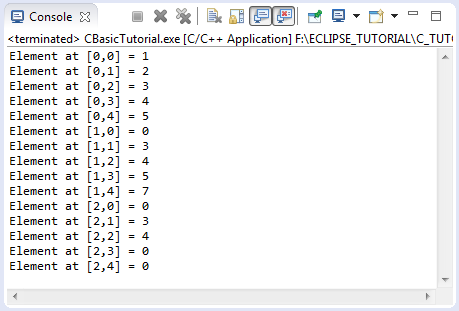## 12- Pointer

A pointer is a variable whose value is the address of another variable, i.e., direct address of the memory location. Like any variable or constant, you must declare a pointer before you can use it to store any variable address. The general form of a pointer variable declaration is:
```type *variable-name;
```
Example:
```// Declare a variable type of int.
int var = 120;

// Declare a pointer (point to address of type int).
int *ip;

// Assign value for ip (Point to address of 'var')
ip = &var;

// Using * to access the value of variable that pointer point to.
int var2 = *ip;
```
PointerExample.cpp
```#include <stdio.h>

int main() {

// Declare a variable
int var = 120;

// Declare a pointer.
int *ip;

// Assign the address of 'var' to pointer.
ip = &var;

// Print out the address of 'var' variable.
printf("Address of var variable: %x \n", &var);

// Print out address stored in 'ip' variable.
printf("Address stored in ip variable: %x \n", ip);

// Access the value of the variable that the pointer is pointing to.
printf("Value of *ip variable: %d\n", *ip);

fflush (stdout);

int var2 = *ip;

return 0;

}
```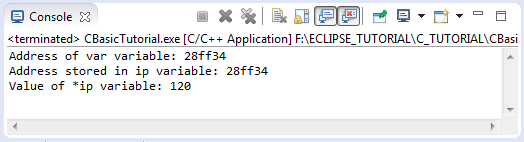### 12.1- NULL Pointer

NULL is a constant predefined in some libraries of C. When you declare a pointer without assigning a specific value for it, it will point to a random memory areas. In some situations you can declare pointer and directly assign NULL to it. Let's look at an example:
NULLPointerExample.cpp
```#include <stdio.h>

int main() {

// Declare a pointer.
// Do not assign any value for this pointer.
// It randomly pointed to certain memory region.
int *pointer1;

// Print out the address of pointer1.
printf("Address of pointer1 is %x \n", pointer1);

// Check if pointer1 is not NULL.
if (pointer1) {
// Print out the value, that the pointer1 point to.
printf("Value of *pointer1 is %d \n", *pointer1);
}

// Declare a pointer.
// Point to nothing (NULL).
int *pointer2 = NULL;

// Print out address of pointer2.
printf("Address of pointer2 is %x \n", pointer2);

// If pointer2 is NULL then print out message.
if (!pointer2) {
printf("pointer2 is NULL");
} else {
// NOTE:
// will receive an error and stop the program.
printf("Value of *pointer2 is %d \n", *pointer2);
}

fflush (stdout);

return 0;
}
```
Running the example: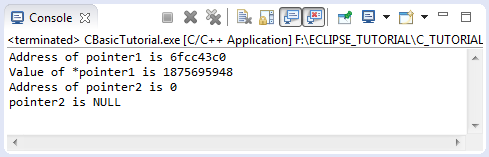## 13- Function

• TODO

#### View more Tutorials:

Maybe you are interested

These are online courses outside the o7planning website that we introduced, which may include free or discounted courses.

•Learn C++ Programming The Easy Way
•Windows 10 C++ App Development for Startups - C++ Simplified
•C++ For Absolute Beginners : The Starter Guide
•Beginning Test Driven Development in C++
•*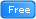* C++, Short and Sweet, Part 1
•Modern OpenGL C++ 3D Game Tutorial Series & 3D Rendering
•Learn C++ Programming Mini Course - Power of Animation
•Concepts of Object Oriented Programming with C++
•Learn C++ by Creating
•c++ Programming for beginners - From Zero to Hero in c++
•Byte-Sized-Chunks: Operator Overloading in C++
•Learn the basics of C++ fast! Learn how to Develop Software
•Learn and Understand C++
•Learn C++ Game Development
•Start With C / C++ and Object Oriented Programming